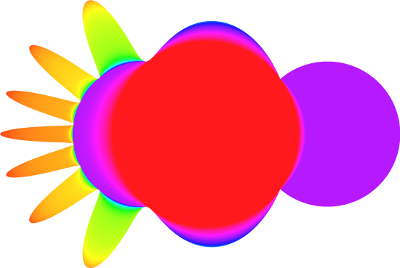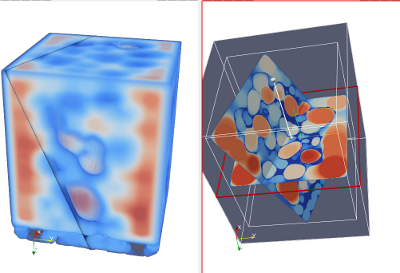DGtal  1.1.0
Tutorial: Volumetric analysis and Granulometry

The objective of this tutorial is to perform some elementary volumetric analysis of 3D objects. With this tutorial, you will experiment the following DGtal features

Please use the following template file for this tutorial: volDTGranulo-template.cpp

# Introduction

When analyzing 3D porous material, the granulometric function plays an important role for shape description. For short, given a shape $$\mathcal{X}$$, the granulometric function at a point $$x\in \mathcal{X}$$ is given by:

$g (x) = \max \{ r~|~ \forall c\in\mathcal{X}, x\in B(c,r)\text{ and }\forall B(c,r)\subseteq \mathcal{X}\}$

$$B(c,r)$$ being an Euclidean ball with center $$c$$ and radius $$r$$.Granulometry function on a 2D shapeGranulometry function on Al objectGranulometry function on

interior/exterior of a porous material (snow sample, courtesy of GAME-CNRM/CEN and ESRF) interior/exterior of a porous material (snow sample, courtesy of GAME-CNRM/CEN and ESRF)

To compute the granulometric function, a classical approach is to first consider the medial axis of $$\mathcal{X}$$ (the medial axis can be roughly defined as the set of maximal balls contained in the shape).

In this tutorial, we will implement a first naive approach from distance transformation values. The distance transformation is a function defined by

$DT (x) = \min \{ d(x,\hat{x}~|~ x\in\mathcal{X}\text{ and }\hat{x}\in \mathbb{R}^n\setminus \mathcal{X}\}$

for some metric $$d$$.

Hence, instead of checking all Euclidean balls inside $$\mathcal{X}$$, we can just consider the digital balls $$B(x, DT(x))$$ for all $$x\in\mathcal{X}$$ (in this case, $$d$$ is the $$l_2$$ Euclidean norm).

# Import volumetric image an visualization

Let start with volumetric image loading. First of all, create a source file with the following includes (or copy the volDTGranulo-tempate.cpp source file):

#include "DGtal/base/Common.h"
#include "DGtal/helpers/StdDefs.h"
#include "DGtal/images/ImageContainerBySTLVector.h"
#include "DGtal/io/writers/VolWriter.h"
#include "DGtal/images/imagesSetsUtils/SimpleThresholdForegroundPredicate.h"
#include "DGtal/geometry/volumes/distance/DistanceTransformation.h"
#include "DGtal/shapes/implicit/ImplicitBall.h"
#include "DGtal/base/BasicFunctors.h"
1. Define a type (typedef) for the image container you want to use (ImageContainerBySTLVector, ImageContainerBySTLMap, ....)
2. Use the VolReader to load a volumetric file and store it into a previously defined image type.

Once you have imported the volumetric file, you can check its geometry with the internal Viewer3D visualization tool. First of all, create a new viewer instance using the following code:

//Create a new viewer instance
QApplication application(argc,argv);
Viewer3D<> viewer;
viewer.show();
Note
Please have a look to Display3D: a stream mechanism for displaying 3D DGtal objects for more details on DGtal viewer.

At this point, just send to the viewer the grid points with strictly positive values.

# Distance Transformation

1. First of all, have a look to the DistanceTransformation class and check the template type you have to specified to this class to create an instance.
2. As you may have seen, one of the input is a binary predicate which characterizes the shape (model of concepts::CPointPredicate concept). Use the functors::SimpleThresholdForegroundPredicate to create a point predicate from an image and a thresold.
3. Now, create a distance map from this point predicate and an Eucildean $$l_2$$ norm.
4. Create a new Viewer3D instance and visualize the distance map values. Two things would be helpful:
• If you want to add a clipping plane to the viewer, a simple object has been defined in DGtal:
viewer << DGtal::ClippingPlane(1,0,0,-10.1);
• The following code will create a nice colormap to map DT values to colors.
//Create the colormap from the maximal distance value
DT::Value maxDT = (*boost::first_max_element(distancemap.constRange().begin(),
distancemap.constRange().end()));

# Granulometric function

As discussed in the introduction, we will implement a very naive version of the granulometric function extraction. The main idea is to construct an image storing the $$g$$ function. The algorithm is the following one:

• Initiaize the map $$g$$ with 0 at each point
• For each grid points $$x$$ in the object
• Create a Ball centered at $$x$$ with radius $$DT(x)$$
• Iteratate over all points $$y$$ in this ball
• If the value $$g(y)$$ is less than $$DT(x)$$, set $$g(y)=DT(x)$$
1. Create an empty image $$g$$ with the same size as the input vol file.
2. Create a first ball (e.g. centered in the middle of the image) using the ImplicitBall class. This class creates an implicit ball such that the () operator is posivive at a point if the point is inside the shape (model of CBoundedEuclideanShape). A basic way to scan grid points inside an ImplicitBall is to construct a Domain from its bounding box, then you scan all points in this domain and check the operator() sign to decide if the point is inside or not.
Note
More advanced digitization processes are given by GaussDigitizer.
1. Implement the above mentioned granulometric function algorithm: at each point of the DT map, create a ball and check its interior point to update the function $$g$$.
2. Again, use a new Viewer3D object to visualize the granulometric function.
3. Export the final map to a vol file. In this case, consider the VolWriter class (granuloImage is the name of image structure storing the $$g$$ map):
VolWriter<Image, CastFunctor<unsigned char> >::exportVol("granulo.vol", imageGranulo);
1. What is the computational cost of this naive approach for a shape defined in a $$N^3$$ domain ?

# Conclusion

You can check volDTGranulo.cpp to get some answers to the above mentioned questions.

Several optimizations can be developed. For example, before constructing the granulometric function, one can use medial axis extraction to drastically reduce the number of balls to scan. Then, sorting the balls by their radii may be helpful to implement fast propagation based granulometric/thickness function from the PowerMap (see for instance ).

DGtal::Color::Green
static const Color Green
Definition: Color.h:401
DGtal::Color::Yellow
static const Color Yellow
Definition: Color.h:406
DGtal::Color::Blue
static const Color Blue
Definition: Color.h:403
DGtal::Color::Red
static const Color Red
Definition: Color.h:400
DGtal::ClippingPlane
Class for adding a Clipping plane through the Viewer3D stream. Realizes the concept CDrawableWithView...
Definition: DrawWithDisplay3DModifier.h:157
Value
double Value
Definition: testSimpleRandomAccessRangeFromPoint.cpp:38